# Introduction

A seminal paper by John Rick some 30 years ago (1987) first introduced the idea of using the frequency of archaeological radiocarbon dates through time as a proxy for highs and lows in human population dynamics. The increased availability of large collection of archaeological (especially anthropogenic) radiocarbon dates have dramatically pushed this research agenda forward in recent years. New case studies from across the globe are regularly being published, stimulating the development of new techniques to tackle specific methodological and interpretative issues.

rcarbon is an R package for the analysis of large collection of radiocarbon dates, with particular emphasis on the “date as data” approach pioneered by Rick (1987). It offers basic calibration functions as well as a suite of statistical tests for examining aggregated calibrated dates, generally referred to as summed probability distributions of radiocarbon dates (SPDs, or sometimes SPDRDs).

## Installing the rcarbon package

Stable versions of the rcarbon package can be directly installed from CRAN (using the command install.packages("rcabon")), whilst the development version can be installed from the github repository using the following command (the function requires the devtools package):

devtools::install_github("ahb108/rcarbon")

Notice that the development version can be unstable, so for general use we recommend the stable CRAN version

# Calibrating $$^{14}$$C Dates

Single or multiple radiocarbon dates can be calibrated using the calibrate() function, which uses the probability density approach (Stuiver and Reimar 1993, Van Der Plicht 1993, Bronk Ramsey 2008) implemented in most calibration software (e.g. OxCal) as well as in other R packages (especially Bchron, which also provides age-depth modelling for environmental cores with radiocarbon dates and experimental options for aggregating dates via Gaussian mixtures).

The example below calibrates a sample with a $$^{14}$$C Age of 4200 BP and an error of 30 years using the intcal13 calibration curve:

x <- calibrate(x=4200,errors=30,calCurves='intcal13')

The resulting object of class CalDates can then be plotted using the basic plot() function (in this case highlighting the 95% higher posterior density interval):

plot(x,HPD=TRUE,credMass=0.95)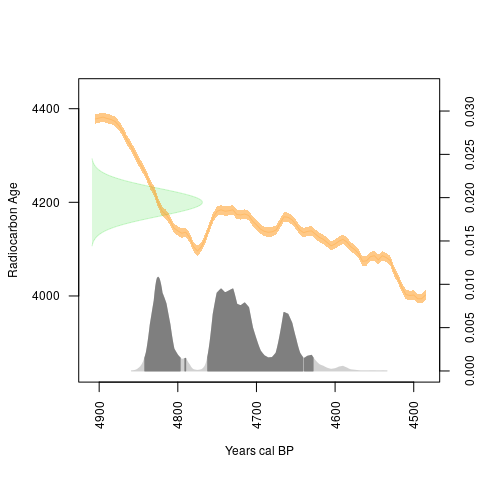Multiple dates can be calibrated by supplying a vector of numerical values, and the summary() function can be used to retrieve one and the two sigma ranges as well as the median calibrated date:

xx <- calibrate(x=c(5700,4820,6450),errors=c(30,40,40),calCurves='intcal13')
summary(xx)
##   DateID MedianBP OneSigma_BP_1 OneSigma_BP_2 TwoSigma_BP_1 TwoSigma_BP_2
## 1      1     6478  6528 to 6521  6508 to 6437  6596 to 6595  6564 to 6407
## 2      2     5527  5599 to 5580  5528 to 5483  5642 to 5628  5615 to 5566
## 3      3     7367  7422 to 7411  7397 to 7328  7430 to 7287      NA to NA
##   TwoSigma_BP_3
## 1      NA to NA
## 2  5562 to 5470
## 3      NA to NA

The calibrate() function can also be executed in parallel by specifying the number of cores using the argument ncores.

Calibration can be done with different curves. The following example is for a marine sample with $$\Delta R = 340\pm20$$:

x <- calibrate(4000,30,calCurves='marine13',resOffsets=340,resErrors=20)
plot(x,HPD=TRUE,calendar="BCAD") #using BC/AD instead of BP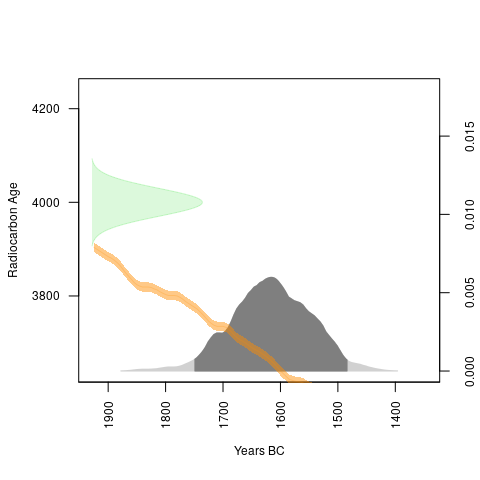Users can also supply their own custom calibration curves. The example below uses a mixed marine/terrestrial curve generated using the mixCurves() function:

#generate 70% terrestrial and 30% marine curve
myCurve <- mixCurves('intcal13',p=0.7,resOffsets=340,resErrors=20)
plot(calibrate(4000,30,calCurves=myCurve))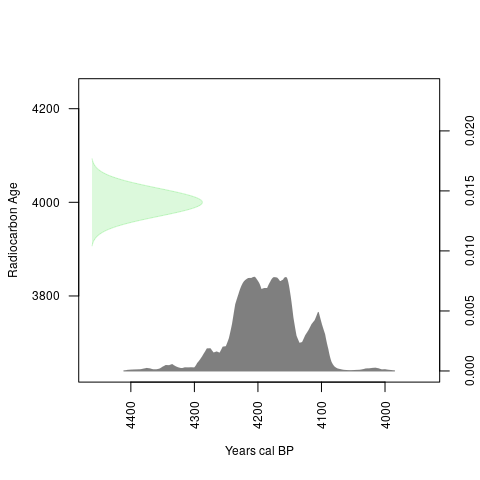### Normalisation

By default, calibrated probabilities are normalised so the total probability is equal to one, in step with most other radiocarbon calibration software. However, Weninger et al (2015) argue that when dates are aggregated by summation, this normalisation process can generate artificial spikes in the resulting summed probability distributions (SPDs) coinciding with steeper portions of the calibration curve. By specifying normalised=FALSE in calibrate() it is possible to obtain non-normalised calibrations. Using normalised or non-normalised calibrations does not have an impact on the shape of individual calibrated probability distribution, but does influence the shape of SPDs, so we suggest that at minimum a case study should explore whether its results differ much when normalised versus unnormalised dates are used.

# Aggregating $$^{14}$$C Dates: Summed Probability Distributions (SPD)

The function spd() aggregates (sums) calibrated radiocarbon dates within a defined chronological range. The resulting object can then be displayed using the plot() function. The example below uses data from the EUREOVOL project database (Manning et al 2016) which can be directly accessed within the package.

data(euroevol)
DK=subset(euroevol,Country=="Denmark") #subset of Danish dates
DK.caldates=calibrate(x=DK$C14Age,errors=DK$C14SD,calCurves='intcal13',ncores=3) #running calibration over 3 cores
DK.spd = spd(DK.caldates,timeRange=c(8000,4000))
plot(DK.spd)
plot(DK.spd,runm=200,add=TRUE,type="simple",col="indianred",lwd=2,lty=2) #using a rolling average of 200 years for smoothing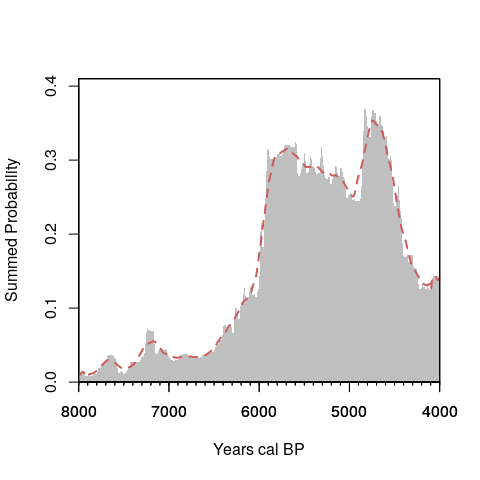It is also possible to limit the display of the SPD to a particular window of time and/or use a ‘BC/AD’ timescale:

# show SPD between 6000 and 4000 BC
plot(DK.spd,calendar='BCAD',xlim=c(-6000,-4000))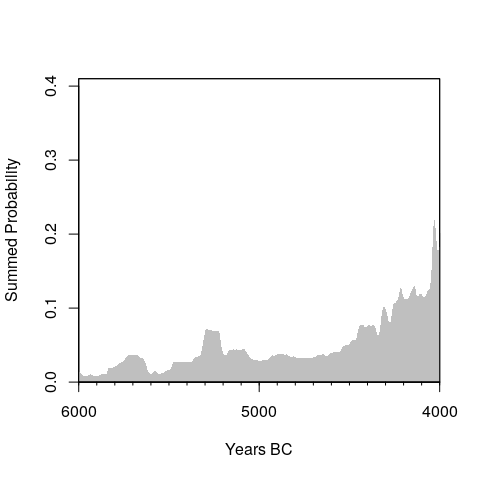# show SPD between 7000 and 5000 BP
# plot(DK.spd,calendar='BP',xlim=c(7000,5000)) 

## Binning

SPDs can be potentially biased if there is strong inter-site variability in sample size, for example where one well-resourced research project has sampled one particular site for an unusual number of dates. This might generate misleading peaks in the SPD and to mitigate this effect it is possible to create artificial bins, a local SPD based on samples associated with a particular site and close in time that is divided by the number of dates in the bin or to the average SPD (in case of non-normalised calibration). Dates are assigned to the same or different bins based on their proximity to one another in (either $$^{14}$$C in time or median calibrated date) using hierarchical clustering with a user-defined cut-off value (using the hclust() function and the argument h) and this binning is implemented by the binPrep() function. The code below illustrates an example using a cut-off value of 100 years:

DK.bins = binPrep(sites=DK$SiteID,ages=DK$C14Age,h=100)
# DK.bins = binPrep(sites=DK$SiteID,ages=DK.caldates,h=100) #using median calibrated date The resulting object can then be used as an argument for the spd() function: DK.spd.bins = spd(DK.caldates,bins=DK.bins,timeRange=c(8000,4000)) plot(DK.spd.bins)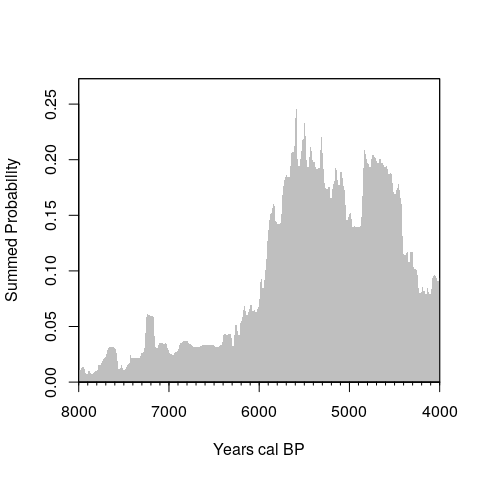The selection of appropriate cut-off values have not been discussed in the literature (Shennan et al 2013 uses a value of 200 years but their algorithm is slightly different). From a practical point a “bin” represents an “phase” or episode of occupation, but clearly this is problematic definition in case of a continuous occupation. The binning process should hence be used with caution, and its implication explored via a sensitivity analysis. The function binsense() enables a visual assessment of how different cut-off values can modify the shape of the SPD. The example below explores 6 different values and show how the highest peak in the SPD changes as function of h but the overall dynamics remains essentially the same. binsense(x=DK.caldates,y=DK$SiteID,h=seq(0,500,100),timeRange=c(8000,4000))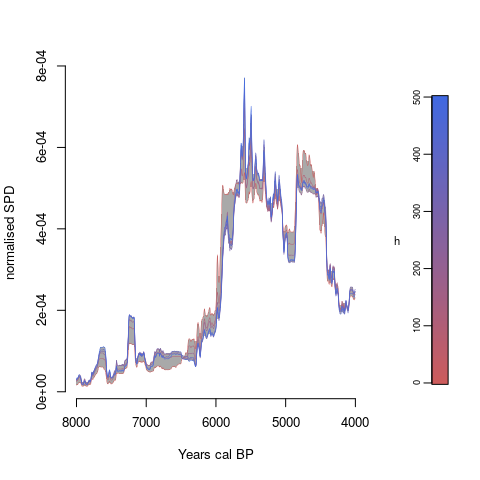### Visualising Bins

The location (in time) of individual bins can be shown by using the binMed() and the barCodes() functions. The former computes the median date from each bin whilst the latter display them as vertical lines on an existing SPD plot.

Dk.bins.med=binMed(x = DK.caldates,bins=DK.bins)
plot(DK.spd.bins,runm=200)
barCodes(Dk.bins.med,yrng = c(0,0.01))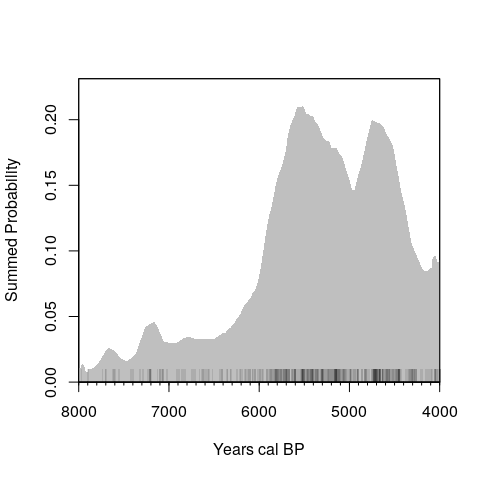# Hypothesis Testing

The shape of empirical SPDs can be affected by a host of possible biases including taphonomic loss, sampling error, and the shape of the calibration curve. One way to approach this problem is to assess SPDs in relation to theoretical expectations and adopt a hypothesis-testing framework. rcarbon provides several functions for doing this.

## Testing against theorethical growth models

Shennan et al 2013 (Timpson et al 2014 for more detail and methodological refinement) introduced a Monte-Carlo simulation approach consisting of a three stage process: 1) fit a growth model to the observed SPD, for example via regression; 2) generate random samples from the fitted model; and 3) uncalibrate the samples. The resulting set of radiocarbon dates can then be calibrated and aggregated in order to generate an expected SPD of the fitted model that takes into account idiosyncrasies of the calibration process. This process can be repeated $$n$$ times to generate a distribution of SPDs (which takes into account the effect of sampling error) that can be compared to the observed data. Higher or lower than expected density of observed SPDs for a particular year will indicate local divergence of the observed SPD from the fitted model, and the magnitude and frequency of these deviations can be used to assess the goodness-of-fit via a global test. rcarbon implements this routine with the function modelTest(), which enables testing against exponential, linear, uniform, and user-defined custom models. The script below shows an example with the Danish SPD fitted to an exponential growth model:

nsim = 100
expnull <- modelTest(DK.caldates, errors=DK$C14SD, bins=DK.bins, nsim=100, timeRange=c(8000,4000), model="exponential",runm=100) We can extract the global p-value from the resulting object which can also be plotted. plot(expnull)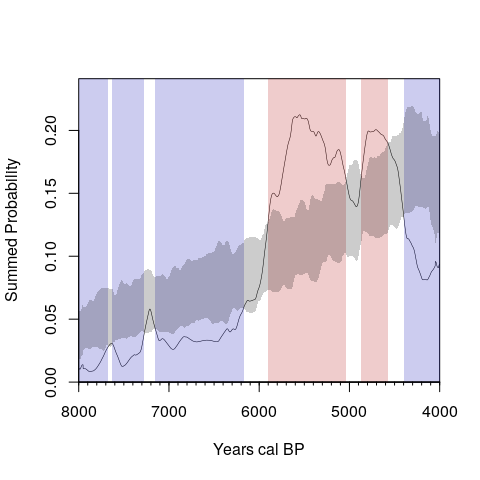expnull$pval #global p-value
##  0.00990099

The grey shaded region represents a critical envelope encompassing the middle 95% of the simulated SPDs, with red and blue regions highlighting portions of the SPD where positive and negative deviations are detected. Further details can be extracted using the summary() function:

summary(expnull)
## 'modelTest()' function summary:
##
## Number of radiocarbon dates: 699
## Number of bins: 443
##
## Statistical Significance computed using 100 simulations.
## Global p-value: 0.0099.
##
## Signficant positive local deviations at:
## 5904~5038 BP
## 4867~4576 BP
##
## Signficant negative local deviations at:
## 8000~7670 BP
## 7626~7272 BP
## 7152~6173 BP
## 4398~4000 BP

### Testing against custom growth models

The modelTest() functions can also be used to test user-defined theoretical growth models. The example below fits a logistic growth model (using the nls() function).

# Generate a smoothed SPD
DK.spd.smoothed = spd(DK.caldates,timeRange=c(8000,4000),bins=DK.bins,runm=100)
# Start values should be adjusted depending on the observed SPD
logFit <- nls(PrDens~SSlogis(calBP, Asym, xmid, scale),data=DK.spd.smoothed$grid,control=nls.control(maxiter=200),start=list(Asym=0.2,xmid=5500,scale=-100)) # Generate a data frame containing the fitted values logFitDens=data.frame(calBP=DK.spd.smoothed$grid$calBP,PrDens=SSlogis(input=DK.spd.smoothed$grid$calBP,Asym=coefficients(logFit),xmid=coefficients(logFit),scal=coefficients(logFit))) # Use the modelTest function (returning the raw simulation output - see below) LogNull <- modelTest(DK.caldates, errors=DK$C14SD, bins=DK.bins,nsim=100,
timeRange=c(8000,4000), model="custom",predgrid=logFitDens, runm=100, raw=TRUE)
# Plot results
plot(LogNull)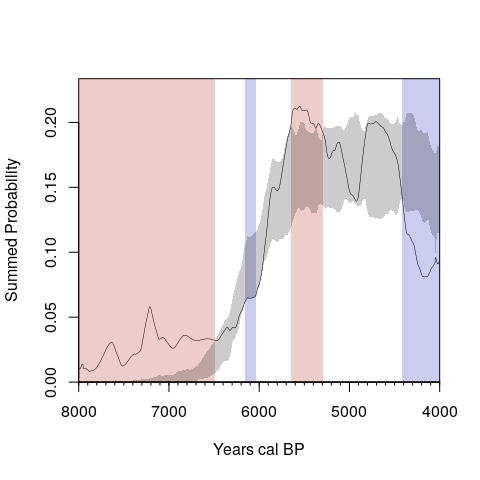# Retrieve p-values
LogNull$pval ##  0.00990099 ### Point-to-point Test The modelTest() function does not discern whether the observed difference between two particular points in time are significant, as both local and global tests are based on the overall shape of the observed and expected SPDs. The p2pTest() follows a procedure introduced by Edinborough et al (2017) which compares the expected and the observed difference in radiocarbon density between just two user-defined points in time. The example below is based on the Danish subset using a uniform theoretical model. #Fit a Uniform model (the argumet raw should be set to TRUE for p2pTest()) uninull <- modelTest(DK.caldates, errors=DK$C14SD, bins=DK.bins, nsim=100, timeRange=c(8000,4000), model="uniform",runm=100, raw=TRUE)
#Test Difference between 5120 and 4920 cal BP
results=p2pTest(uninull,p1=5120,p2=4920)

Notice that when the arguments p1 and p2 are not supplied p2pTest() displays the SPD and enable users to interactively select the two points on the plotted SPD.

## Comparing empirical SPDs against each other

SPDs are often compared against each other to evaluate regional variations in population trends (e.g.Timpson et al 2015) or to determine whether the relative proportion of different dated materials changes across time. Collard et al (2010) for instance demonstrates that the relative frequency of different kinds of archaeological site has varied over time in Britain, whilst Stevens and Fuller (2012) argue that the proportion of wild versus domesticated crops fluctuated during the Neolithic (see also Bevan et al. 2017). The permTest() function provides a permutation test for comparing two or more SPDs, returning both global and local p-values using similar procedures to modelTest() (first introduced by Crema et al. 2016).

The example below reproduces the analyses of Eastern Mediterranean dates by Roberts et al (2017):

data(emedyd) # load data
cal.emedyd = calibrate(emedyd$CRA,emedyd$Error,ncores=3,normalised=FALSE)
bins.emedyd = binPrep(ages = emedyd$CRA,sites = emedyd$SiteName,h=50)
perm.emedyd=permTest(x=cal.emedyd,marks=emedyd$Region,timeRange=c(16000,9000),bins=bins.emedyd,nsim=100,runm=50) summary(perm.emedyd) par(mfrow=c(3,1)) plot(perm.emedyd,focalm = 1,main="Southern Levant") plot(perm.emedyd,focalm = 2,main="Northern Levant/Upper Mesopotamia") plot(perm.emedyd,focalm = 3,main="South-Central Anatolia")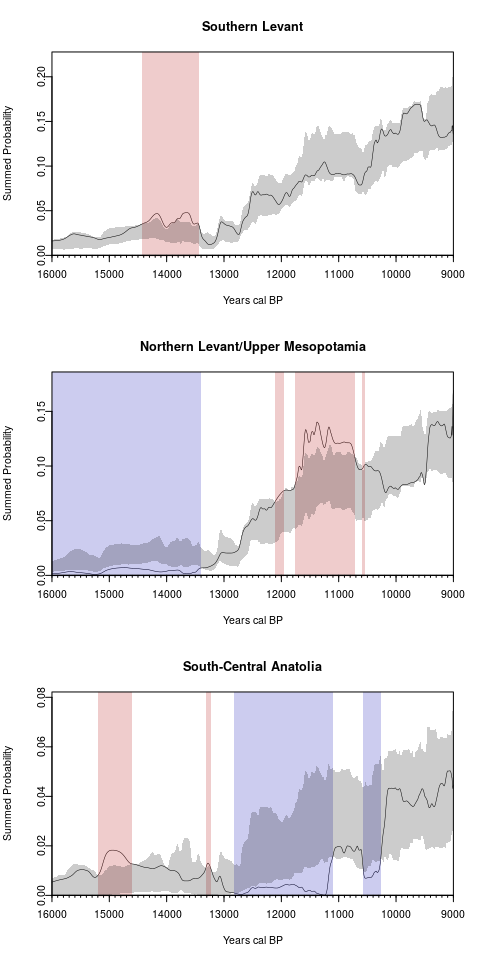## Spatial Analysis When geographic study areas are very large, it becomes inappropriate to assume that there is complete spatial homogeneity in the demographic trajectories of different sub-regions in the study area. At the same time, evaluating such regional divergences is difficult because any increase in spatial scale of a study usually entails also an increase in the heterogeneity of research design and in overall sampling intensity. rcarbon enables an exploration of spatial heterogeneity in the SPDs that is robust to differences in sampling intensity and provides a permutation-based statistical significance framework (for details in the method see Crema et al. 2017). In order to carry out a spatial analysis of aggregate radiocarbon dates, we need calibrated dates, bins, and a SpatialPoints class object containing the site locations: euroevol=subset(euroevol,C14Age<=7200&C14Age>=4200) eurodates <- calibrate(euroevol$C14Age,euroevol$C14SD,normalised=FALSE,verbose=FALSE) eurobins <- binPrep(sites=euroevol$SiteID,ages=euroevol$C14Age,h=200) # Create a data.frame of site locations extracting spatial coordinates sites <- unique(data.frame(id=euroevol$SiteID,lat=euroevol$Latitude,lon=euroevol$Longitude))
rownames(sites) <- sites\$id
sites <- sites[,-1]

# Convert to a SpatialPoints class object:
sp::coordinates(sites) <- c("lon","lat")
sp::proj4string(sites) <- sp::CRS("+proj=longlat +datum=WGS84")

We then need to generate a distance matrix which we will use to define the spatial weighting scheme using the spweights() function. The example below uses a Gaussian decay function (the default) with a bandwidth size of 100 km:

#Compute distance matrix
d <- sp::spDists(sites,sites,longlat=TRUE)
#Compute spatial weights
w <- spweights(d,h=100)

The core function SPpermTest() compares the observed and the expected geometric growth rates rather than the raw SPD. Thus we need to define the break points of our chronological blocks and of course the overall time range of our analysis. In this case we examine sequences blocks of 500 years.

breaks <- seq(8000,5000,-500) #500 year blocks
timeRange <- c(8000,5000) #set the timerange of analysis in calBP, older date first

The function spd2gg() can be used to calculate and visualise the growth rates for specific sequence of blocks.

eurospd = spd(x = eurodates,bins=eurobins,timeRange = timeRange)
plot(spd2gg(eurospd,breaks = breaks))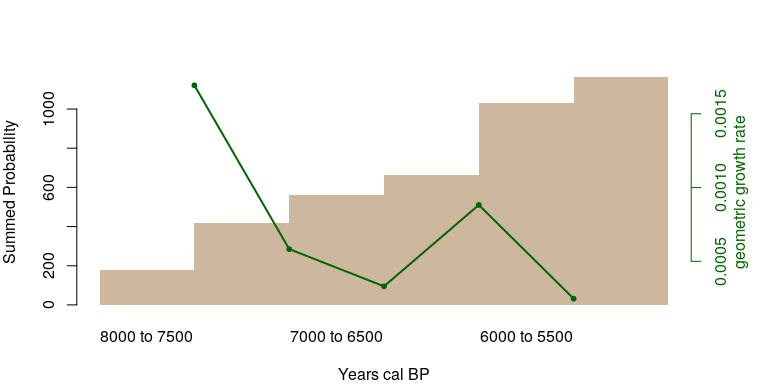In this case the pan-regional trend shows a positive but declining growth rates through time, with the exception of the transition from to 6500-6000 to 6000-5500 cal BP when the rate increases slightly.

In order examine whether this dynamics is observed across the study region we execute our permutation test with the SPpermTest() function:

eurospatial <- SPpermTest(calDates=eurodates,bins=eurobins,timeRange=timeRange,
locations=sites,permute="locations",nsim=1000,
breaks=breaks,spatialweights=w,ncores=3) 

The output of the function has its own plot() method which provides various ways to display the outcome. The function plots only the point locations, so it is often convenient to load a separate base map. The example below uses the rworldmap package:

library(rworldmap)
base <- getMap(resolution="low") #extract basemap
#extract bounding coordinates of the site distribution
xrange <- bbox(sites)[1,]
yrange <- bbox(sites)[2,]

The plot function requires the definition of an index value (a numerical integer representing the i-th transition (thus index=1 means first transition, in this case the transition from the time block 8000-7500 to the time block 7500-7000 calBP), and an option argument, which indicates what needs to be plotted (either the results of the statistical tests or the local estimates of geometric growth rates). The scripts below examines the transition when the declining growth rate exhibits a short reversion (i.e. 6500-6000 to 6000-5500 cal BP).

The plot function requires the definition of an index value (a numerical integer representing the i-th transition (thus index=1 means first transition, in this case the transition from the time block 8000-7500 to the time block 7500-7000 calBP), and an option argument, which indicates what needs to be plotted (either the results of the statistical tests or the local estimates of geometric growth rates). The scripts below examines the transition when the declining growth rate exhibits a short reversion (i.e.6500-6000 to 6000-5500 cal BP).

## Spatial Permutation Test for Transition 4
par(mar=c(1,1,4,1),mfrow=c(1,2))
plot(base,col="antiquewhite3",border="antiquewhite3",xlim=xrange,ylim=yrange,main="6.5-6 to 6-5.5 kBP \n (Test Results)")
plot(eurospatial,index=4,option="raw",add=TRUE,breakRange=c(-0.005,0.005),legend=TRUE,legSize=0.7,location="topleft")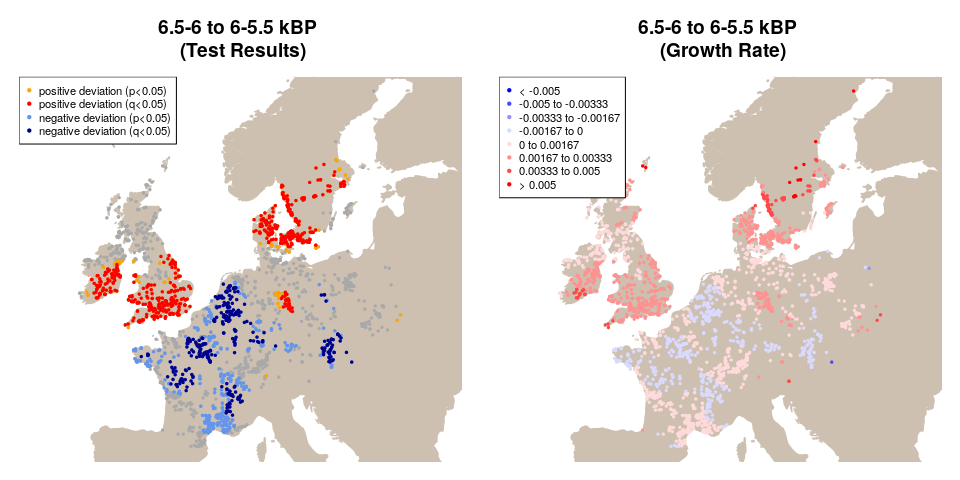The two figures shows signficant spatial hetorogeneity in growth rates. Southern Ireland, Britain, and the Baltic area all exhibit positive growth, while most of France is associated with negative deviations from the pan-regional model. Given the large number of site locations and consequent inflation of type I error, SPpermTest() calculates also the false discovery rate (q-values) using the p.adjust() function with method="fdr". A q-value of 0.05 implies that 5% of the results that have a q-value below 0.05 are false positives.